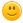# what is 2444 +244444995

171 views
asked Mar 9, 2013 in Math

answered Jul 5, 2015 by (1,620 points)
The answer is 244447439.I agree with whizkid on not only the answer,but the way whizkid did it.I love math :P
+1 vote
answered Jun 9, 2015 by (424,180 points)
244,447,439
answered Jul 21, 2014 by (424,180 points)
I think you have a calculator on your computer

Or there could be a calc on your place

or also go on tutorials to check how to do it.
answered Mar 17, 2013 by (41,740 points)
Addtitionally, you could also go to KHAN ACADEMY which is an online website. Sal Khan, the creator, makes videos about math, science, and anything basically, that are very easy to follow. You can make you own account and get prizes and badges. He makes Math fun for those who don't like Math. I looove math, hence my name!
answered Mar 17, 2013 by (17,920 points)
To do this, you could use a calculator, but I'm going to tell you how to do it simply.

answered Mar 10, 2013 by (41,740 points)
edited Apr 2, 2017

I can buy a calculator for you if you want.Whizkid is right. Just line up the digits and add the numbers as if they were single-digits.

commented Jul 21, 2013 by (32,490 points)
How can you buy a calculator for him?
answered Mar 9, 2013 by (65,700 points)
edited Mar 10, 2013

You can punch it in a calculator, or do it by hand. It helps to put in the commas after every 3 digits for tracking things

 +      2,444 244,444,995 -------------

Make sure to line up to the right so the same decimal units are over each other and add each column. Remember to carry over the 10's to the left.

5+4=9  put down 9

9+4 = 13, so put down 3 and carry the 1

9+4+1=14, put down 4 and carry the 1

4+2 +1 = 7 put down 7 (no carry)

The rest is just added to 0's so drop them down.

Total= 244,447,439